# Texas Go Math Grade 7 Lesson 9.3 Answer Key Area of Circles

Refer to our Texas Go Math Grade 7 Answer Key Pdf to score good marks in the exams. Test yourself by practicing the problems from Texas Go Math Grade 7 Lesson 9.3 Answer Key Area of Circles.

## Texas Go Math Grade 7 Lesson 9.3 Answer Key Area of Circles

Essential Question
How do you find the area of a circle?

Texas Go Math Grade 7 Lesson 9.3 Explore Activity 1 Answer Key
You can use what you know about circles and π to help find the formula for the area of a circle.
Step 1
Use a compass to draw a circle and cut it out.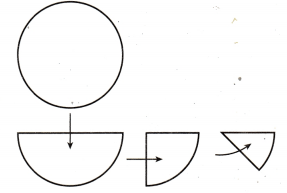Step 2
Fold the circle three times as shown to get equal wedges.
Step 3
Unfold and shade one-half of the circle.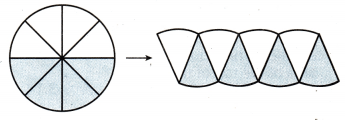Step 4
Cut out the wedges, and fit the pieces together to form a figure that looks like a parallelogram.

The base and height of the parallelogram relate to the parts of the circle.
base b =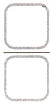the circumference of the circle, or ______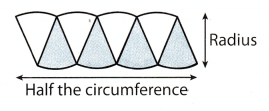height h = the ___________ of the circle, or ______
To find the area of a parallelogram, the equation is A = ___
To find the area of the circle, substitute for b and h in the area formula.Reflect

Go Math Grade 7 Pdf Practice and Homework Lesson 9.3 Question 1.
How can you make the wedges look more like a parallelogram?
In order to make a parallelogram for the wedges of the circle, cut one wedge into half. Then re-arrange the
wedges alternating each wedge to form a parallelogram. The two halves of one wedge will go on each end side.
This will form a rectangle, a kind of parallelogram.Making a circle into a parallelogram.

Finding the Area of a Circle

Area of circle
The area of a circle is equal to π times the radius squared.
A = πr2Remember that area is given in square units.

Example 1
A biscuit recipe calls for the dough to be rolled out and circles to be cut from the dough. The biscuit cutter has a radius of 4 cm. Find the area of the biscuit once it is cut. Use 3.14 for π.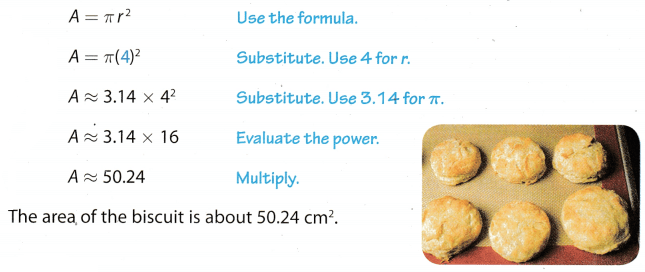Reflect

Question 2.
Compare finding the area of a circle when given the radius with finding the area when given the diameter.
The area of the circle when given the radius is
A = πr2
Since the diameter is twice the radius, the formula for the area of a circle, when given the diameter, is
A = πr2 Substitute $$\frac{d}{2}$$ for r.Question 3.
Why do you evaluate the power in the equation before multiplying?
Because the power refers to the radius only, not the number π.

A circular pool has a radius of 10 feet. What is the area of the pool? Use 3.14 for π. ______
r = 10 feet
Use the formula for the area of the circle, cause the pool is circular.
A = πr(r)2 Substitute 10 for r, and 3.14 for π.
A ≈ 3.14(2)2
A ≈ 3.14 . 100
A ≈ 314
The area of the pool is about 314 feet

Explore Activity 2
Finding the Relationship between Circumference and Area
You can use what you know about the circumference and area of circles to find a relationship between them.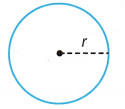Find the relationship between the circumference and area of a circle.The circumference of the circle squared is equal to
___________

Reflect

Question 5.
Does this formula work for a circle with a radius of 3 inches? Show your work.
The radius of the circle is 3 in.
The circumference of the circle squared is equal to
C2 = 4π . A,
where A represents the area of the circle.
First find the area of the circle with the radius of 3 in.
Use the formula for the area of the circle.
A = π(r)2 Substitute 3 for r, and 3.14 for π.
A ≈ 3.14(3)2
A ≈ 3.14 . 9
A ≈ 28.26
The area of the circle is about 28.26 in.
Use the formula for the circumference when given the area
C2 = 4π . A Substitute 28.26 for A and 3.14 for π.
C2 ≈ 4 . 3.14 ∙ 28.26
C2 ≈ 354.94 Root both sides.
$$\sqrt{C^{2}}$$ ≈ $$\sqrt{354.94}$$
The circumference of the circle is 18.84 in.

Let’s see if we get the same result if we use the original formula for circumference
Use the formula for the circumference of the circle.
C = 2πr(r) Substitute 3 for r and 3.14 for π.
C ≈ 23.14 ∙ 3
C ≈ 18.84
The circumference of the circle is 18.84 in.
Hence, we can use both formulas for the circumference of the circle.

The formula works, so we can use both formulas for the circumference of the circle.

Find the area of each circle. Round to the nearest tenth if necessary. Use 3.14 for π. (Explore Activity 1)

Question 1.The diameter of the circle is 14,m.
Use the formula for the area of the circle
A = π(r)2
Since the diameter is twice the radius, the formula for the area of a circle, when given the diameter, isThe area of the circle is about 153.86 m2.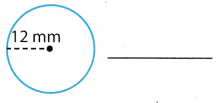The radius of the circle is 12 mm.
Use the formula for the area of the circle.
A = π(r)2 Substitute 12 mm for r, and 3.14 for π.
A ≈ 3.14 ∙ (12 mm)2
A ≈ 3.14 ∙ 144 mm2
A ≈ 452.16 mm2
The area of the circle is about 452.16 mm2.

Question 3.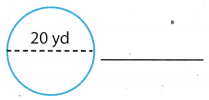The diameter of the circle is 20 yd
Use the formula for the area of the circle
A = π(r)2
Since the diameter is twice the radius, the formula for the area of a circle, when given the diameter, is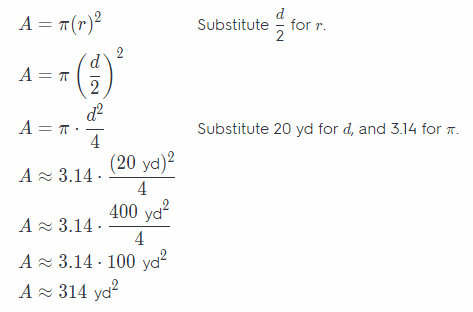The area of the circle is about 314 yd2.

Solve. Use 3.14 for π. (Example 1)

Question 4.
A clock face has a radius of 8 inches. What is the area of the clock face? Round your answer to the nearest hundredth.
The radius of the circle is 8 in.
Use the formula for the area of the circle
A = π(r)2 Substitute 8 in. for r, and 3.14 for π.
A ≈ 3.14. (8 in)2
A ≈ 3.14 ∙ 64 in2
A ≈ 200.96 in2
The area of the circle is about 200.96 in2

Question 5.
A DVD has a diameter of 12 centimeters. What is the area of the DVD? Round your answer to the nearest hundredth.
The diameter of the circle is 12 cm.
Use the formula for the area of the Circle.
A = π(r)2
Since the diameter is twice a radius, the formula for area of a circle, when given the diameter, is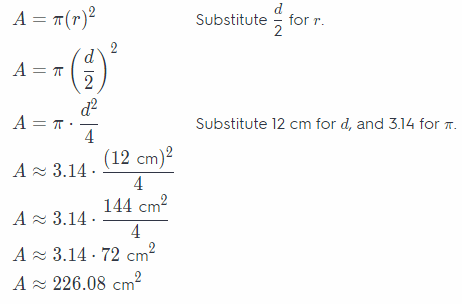The area of the circle is about 226.08 cm2.

Go Math Book 7th Grade Area of a Circle Answer Key Question 6.
A company makes steel lids that have a diameter of 13 inches. What is the area of each lid? Round your answer to the nearest hundredth.
The diameter of the circle is 13 in.
Use the formula for the area of the circle.
A = π(r)2
Since the diameter is twice a radius, the formula for area of a circle, when given the diameter, isThe area of the circle is about 132.66 in2.

Find the area of each circle. Give your answers in terms of π. (Explore Activity 2)

Question 7.
C = 4π
A = ____
Use the formula for the circumference of the circle.Use the formula for area of the circle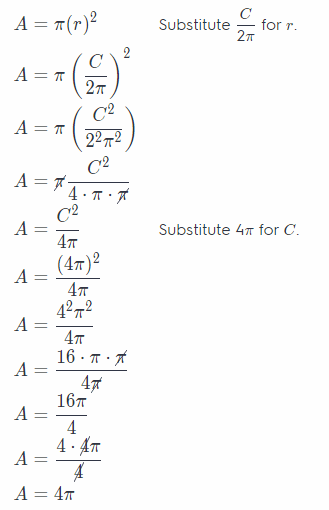Question 8.
C = 12π
A = ___
Use the formula for the circumference of the circle.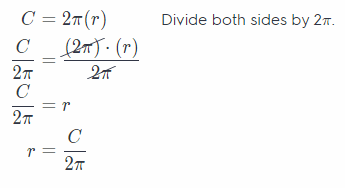Use the formula for area of the circle.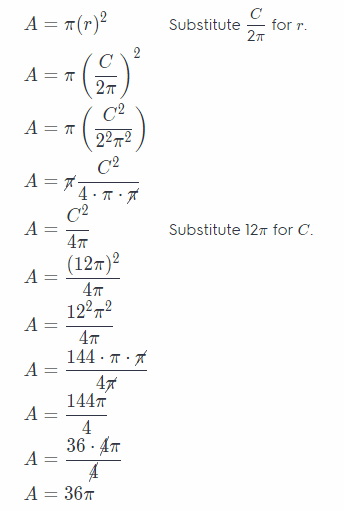Area in Terms of Circumference Answer Key Pdf Grade 7 Question 9.
C = $$\frac{\pi}{2}$$
A = ____________
Use the formula for the circumference of the circle.Use the formula for area of the circle.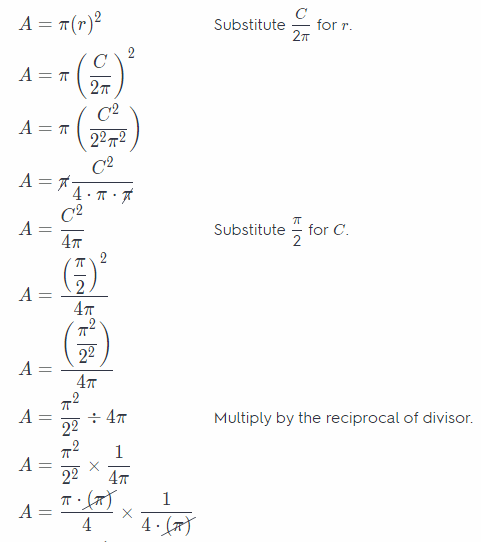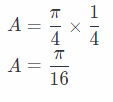Question 10.
A circular pen has an area of 64π square yards. What is the circumference of the pen? Give your answer in terms of π. (Explore Activity 2)
___________
Use the formula for the circumference of the circle.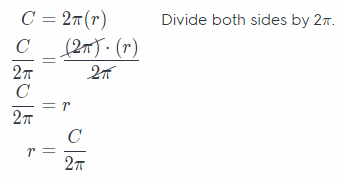Use the formula for area of the circle.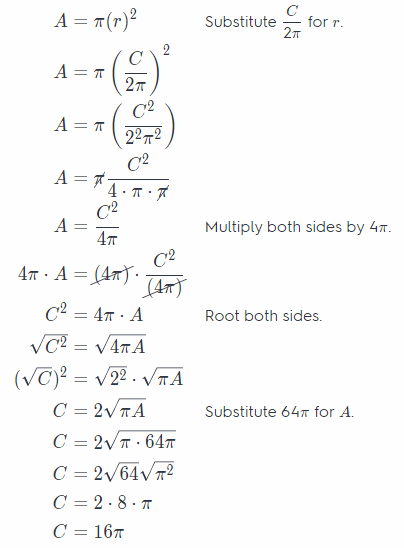Essential Question Check-In

Question 11.
What is the formula for the area A of a circle in terms of the radius r?
A = r2π

Independent Practice

Question 12.
The most popular pizza at Pavone’s Pizza is the 10-inch personal pizza with one topping. What is the area of a pizza with a diameter of 10 inches? Round your answer to the nearest hundredth.
The diameter of the pizza is 10 in.
Use the formula for area of the circle.
Since the radius is half the diameter, formula for the area of the pizza will be
A = π(r)2 Substitute $$\frac{d}{2}$$ for r.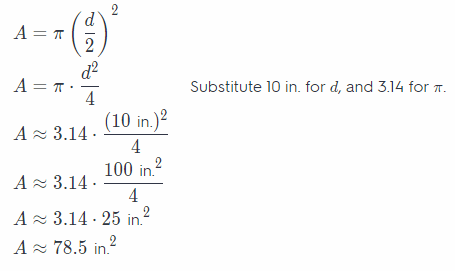The area of the pizza is about 78.5 in.2.

Lesson 9.3 Area of Circles Answer Key Go Math Grade 7 Question 13.
A hubcap has a radius of 16 centimeters, What is the area of the hubcap? Round your answer to the nearest hundredth.The radius of the hubcap is 16 cm.
Use the formula for area of the circle to find area of the hubcap.
A = π(r)2 Substitute 16 cm for r, and 3.14 for π.
A ≈ 3.14(16 cm)2
A ≈ 3.14 256 cm2
A ≈ 803.84 cm2
The area of the hubcap is about 803.84 cm2.

Question 14.
A stained glass window is shaped like a semicircle. The bottom edge of the window is 36 inches long. What is the area of the stained glass window? Round your answer to the nearest hundredth.
The bottom edge of the window represents the diameter of the circle whose half is a window, hence, the area of the window is half of the area of the circle.
Use the formula for area of the circle, where instead of the radius, we place the diameter which is twice the radius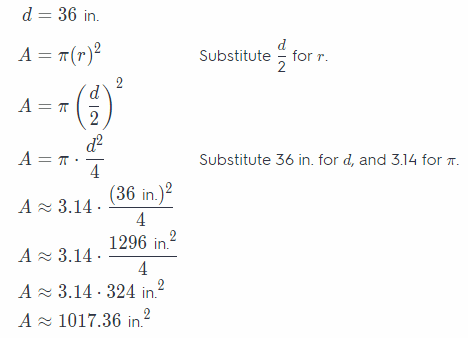The area of the circle is about 1017.36 in.2.
Hence, the area of the window is half of the area of the circle
A ÷ 2 ≈ 1017.36 ÷ 2 ≈ 508.68 in.2
The area of the window is about 508.68 in.2.

Question 15.
Analyze Relationships The point (3, 0) lies on a circle with the center at the origin. What is the area of the circle to the nearest hundredth?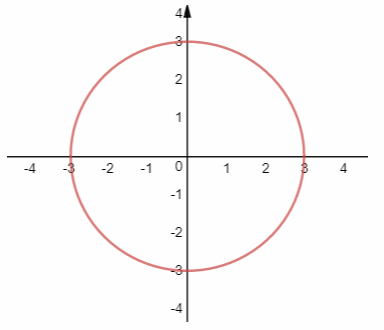As the center of the circle is origin, the distance from it to the point(3, 0) is the radius.
From the diagram above, we see that the radius of the circle is 3.
Use the formula for the area of the circle.
A = π(r)2 substitute 3 for r, and 3.14 for π.
A ≈ 3.14(3)2
A ≈ 3.14 ∙ 9
A ≈ 28.26
The area of the circle is about 28.26

Question 16.
Multistep A radio station broadcasts a signal over an area with a radius of 50 miles. The station can relay the signal and broadcast over an area with a radius of 75 miles. How much greater is the area of the broadcast region when the signal is relayed? Round your answer to the nearest square mile.
We have to find the area of the signal that the station can relay, and the area of the signal that station broadcasts.
The station can relay the signal over an area with a radius of 75 miles, so the area of it will be
A1 ≈ π(r)2 Substitute 75 miles for r, and 3.14 for π.
A1 ≈ 3.14(75 miles)2
A1 ≈ 3.14 ∙ 5625 miles2
A1 ≈17, 662.5 miles2
The area of the signal that the station can relay is about 17, 662.5 miles2.
On the other side, the station broadcasts a signal over an area with a radius of 50 miles, so the area of it will be
A2 = π(r)2 Substitute 50 miles for r, and 3.14 for π.
A2 ≈ 3.14(50 miles)2
A2 ≈ 3.14 ∙ 2500 miles2
A2 ≈ 7 miles
A2 ≈ 7,850 miles2
The area of the signal that the station broadcasts is about 7,850 miles2.
The area of the broadcast region when the signal is relayed, is greater for the difference between the area of the signal that the station can relay, and the area of the signal that station broadcasts.
A1 – A2 ≈ 17,662.5 – 7,850 ≈ 9,812.5 miles2

Question 17.
Multistep The sides of a square field are 12 meters. A sprinkler in the center of the field sprays a circular area with a diameter that corresponds to a side of the field. How much of the field is not reached by the sprinkler? Round your answer to the nearest hundredth.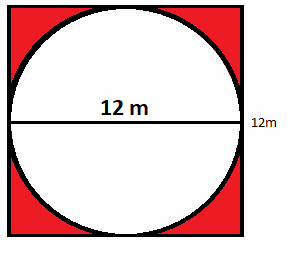The area marked with red color is the area sprinkler does not reach.
To find it, first we have to find the area of the square, and a circular area that a sprinkler sprays.
Use the formula for the area of a square.
A1 = a2 where a represents side of a square. Substitute 12 m for a.
A1 = 12 m2
A1 = 144 m2
The area of the square is 144 m2.
Use the formula for the area of the circle with a diameter same as a square.
Since the radius is half of the diameter, it follows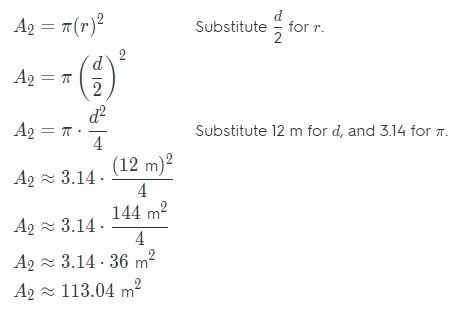The area of the circular area that a sprinkler sprays is about 113.04 m2
From the area of the square subtract the area of the circular area to get the area the sprinkler does not reach.
À1 – A2 ≈ 144 m2 – 113.04 m2 ≈ 30.96 m2
The area the sprinkler does not reach is about 30.96 m2.

Lesson 9.3 Area of Circles Answer Key Go Math Pdf Grade 7 Question 18.
Justify Reasoning A small silver dollar pancake served at a restaurant has a circumference of 2π inches. A regular pancake has a circumference of 4π inches. Is the area of the regular pancake twice the area of the silver dollar pancake? Explain.
Find the area of the small silver dollar pancake and the area of the regular pancake.
First, find the formula for the area of the circle when given circumstance.
Use the formula for the circumference of the circle.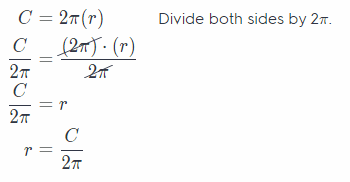Use the formula for area of the circle.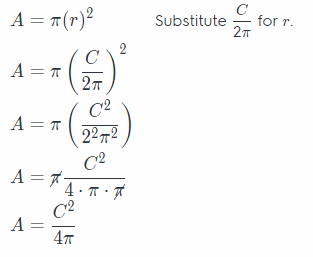The circumference of the small silver dollar pancake is 2π in.
Use the formula above for the area.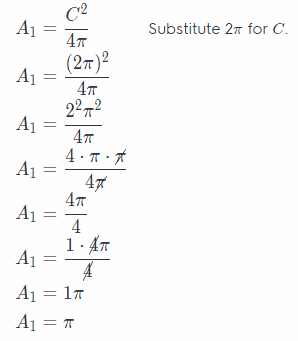The area of the small silver dollar pancake is π in.2.

The circumference of the regular pancake is 4π in.
Use the formula above for the area.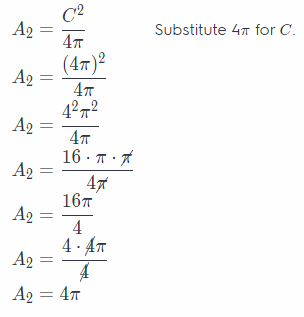The area of the regular pancake is 4π in.2.
A2 – A1 =4π – π = 3π
The area of the regular pancake is more than twice the area of the small silver dollar pancake.

Question 19.
Analyze Relationships A bakery offers a small circular cake with a diameter of 8 inches. It also offers a large circular Cake with a diameter of 24 inches. Does the top of the large cake have three times the area of that of the small cake? If not, how much greater is its area? Explain.
Find the area of the small and large circular cakes.
Use the formula for the area of a circle.
Since the diameter is twice the radius, the formula for the area of a circle when given the diameter is
A = π(r)2 Substitute $$\frac{d}{2}$$ for r.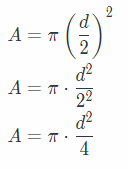Let d1 be the diameter of the larger cake and d2 the diameter of the smaller cake.
d1 = 24 inches
d2 = 8 inches
Use the formula above to find the area of the large cake.The area of the large cake is about 452.16 in.2.
Use the formula above to find the area of the small cake.The area of the small cake is about 50.24 in.2
The top of the large cake have area greater than the area of the small cake for about
A1 – A2 ≈ 452.16 – 50.24 ≈ 401.92 in.2
Hence, the area of the large cake is more than 3 times the area of the small cake.

Question 20.
Communicate Mathematical Ideas You can use the formula A = $$\frac{C^{2}}{4 \pi}$$ to find the area of a circle given the circumference. Describe another way to find the area of a circle when given the circumference.
The other way is to find the radius first Since C = 2πr, we have r = $$\frac{C}{2 \pi}$$. After that, we can find the area by substituting for r in A = πr2.
Determine the radius first with the formula r = $$\frac{C}{2 \pi}$$

Question 21.
Draw Conclusions Mark wants to order a pizza. Which is the better deal? Explain.Hence, a pizza with a diameter of 18 inches for $20 will better deal because in this case will get a larger pizza as compared to a tile pizza with a diameter 12 inch for$10.

Question 22.
Multistep A bear was seen near a campground. Searchers were dispatched to the region to find the bear.
a. Assume the bear can walk in any direction at a rate of 2 miles per hour. Suppose the bear was last seen 4 hours ago. How large an area must the searchers cover? Use 3.14 for π. Round your answer to the nearest square mile. _______
Determine the area the searchers must cover The radius of the area the bear walks is 8 miles since the bear was
last seen 4 hours ago with the rate of 2 miles per hour
A = πr2 Write the formula for the area of a circle
A = (3.14)(8)2 Substitute the values
A = (3.14)(64) Evaluate the exponent
A = 200.96 Multiply the values
A = 201 Round off

b. What If? How much additional area would the searchers have to cover if the bear were last seen 5 hours ago?
Determine the area the searchers must cover. The radius of the area the bear walks is 10 miles since the bear was
last seen 5 hours ago with the rate of 2 miles per hour.
A = πr2 Write the formula for the area of a circle
A = (3.14)(10)2 Substitute the values
A = (3.14)(100) Evaluate the exponent
A = 314 Multiply the values
Determine the additional area the searchers need to cover
314 – 201 = 113
The searchers need to cover an additional 113 square miles.

Texas Go Math Grade 7 Lesson 9.3 H.O.T. Focus on Higher Order Thinking Answer Key

Question 23.
Analyze Relationships Two circles have the same radius. Is the combined area of the two circles the same as the area of a circle with twice the radius? Explain.
The combined area of the two circles, with the same radius, is twice the area of that circle.
Use the formula for the area of the circle.
A = the combined area
A1 the area of the circle
A = 2 ∙ A1
A = 2πr2
The area of the circle with twice the radius is
A = π(2r)2
A = π22r2
A = 4πr2
Hence, the combined area of the two circles with the same radius isn’t the same as the area of the circle with twice

Question 24.
Look for a Pattern How does the area of a circle change if the radius is multiplied by a factor of n, where n is a whole number?
If we multiply the radius by a whole number n, the area of the circle will be
A = π(nr)2
A = πn2r2
Hence, the area of the circle will be n2 times larger.

Question 25.
Represent Real World Problems The bull’s eye on a target has a diameter of 3 inches. The whole target has a diameter of 15 inches. What part of the whole target is the bull’s-eye? Explain.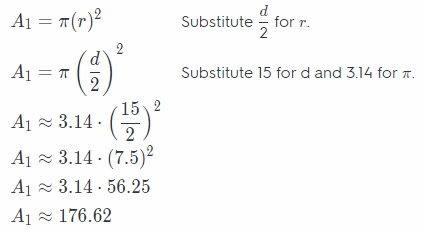$$\frac{A_{1}}{A_{2}}$$ = $$\frac{176.62}{7.06}$$ = 25.01